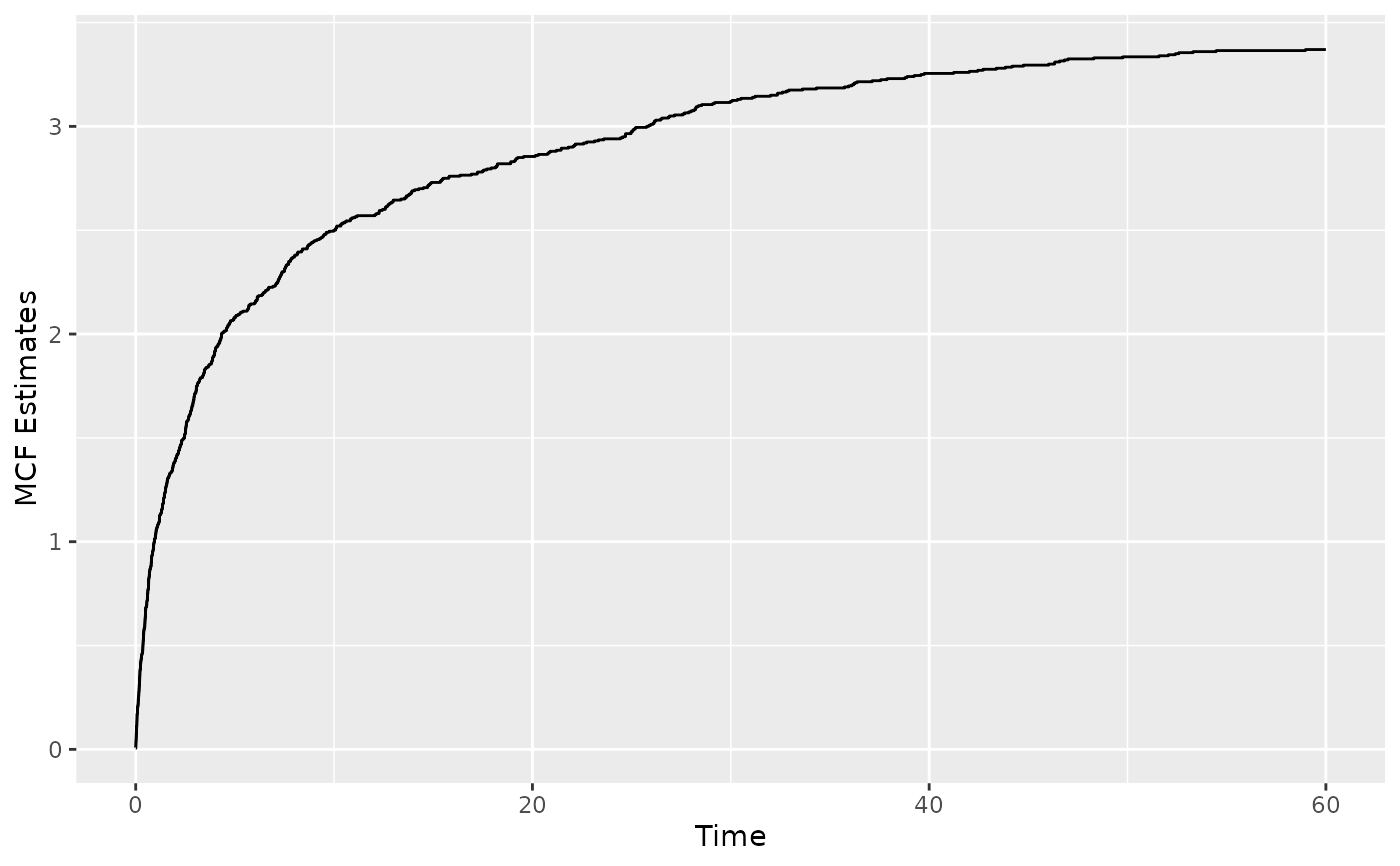Plot the event plot or the mean cumulative function (MCF) from an Recur object.

# S3 method for Recur
plot(
x,
mcf = FALSE,
event.result = c("increasing", "decreasing", "asis"),
event.calendarTime = FALSE,
mcf.adjustRiskset = TRUE,
mcf.conf.int = FALSE,
control = list(),
...
)

## Arguments

x an object of class Recur returned by the Recur() function. See ?Recur for creating Recur objects. an optional logical value indicating whether the mean cumulative function (MCF) will be plotted instead of the event plot. When mcf = TRUE, the mcf is internally called. See mcf for details. an optional character string that is passed to the plotEvents() function as the result argument. See plotEvents. This argument is used to specify whether the event plot is sorted by the subjects' terminal time. The available options are increasingsort the terminal time from in ascending order (default). This places longer terminal times on top. decreasingsort the terminal time from in descending order. This places shorter terminal times on top. nonepresent the event plots as is, without sorting by the terminal times. an optional logical value indicating whether to plot in calendar time. When event.calendarTime = FALSE (default), the event plot will have patient time on the x-axis. an optional logical value that is passed to the mcf() function as the adjustRiskset argument. This argument indicates whether risk set size will be adjusted. If mcf.adjustRiskset = TRUE, subjects leave the risk set after terminal times as in the Nelson-Aalen estimator. If mcf.adjustRiskset = FALSE, subjects remain in the risk set after terminal time. an optional logical value that is passed to the mcf() function as the conf.int argument. See mcf for details. a list of control parameters. See Details. additional graphical parameters to be passed to methods.

## Value

A ggplot object.

## Details

The argument control consists of options with argument defaults to a list with the following values:

xlab

customizable x-label, default value is "Time".

ylab

customizable y-label, default value is "Subject" for event plot and "Cumulative mean" for MCF plot.

main

customizable title, the default value is "Recurrent event plot" when mcf = FALSE and "Sample cumulative mean function plot" when mcf = TRUE.

terminal.name

customizable label for terminal event, the default value is "Terminal event".

recurrent.name

customizable legend title for recurrent event, the default value is "Recurrent events".

recurrent.types

customizable label for recurrent event type, the default value is NULL.

alpha

between 0 and 1, controls the transparency of points.

The xlab, ylab and main parameters can be specified outside of the control list.

Nelson, W. B. (1995) Confidence Limits for Recurrence Data-Applied to Cost or Number of Product Repairs. Technometrics, 37(2): 147--157.

## See also

Recur, plotEvents, mcf

## Examples

data(simDat)
reObj <- with(simDat, Recur(t.start %to% t.stop, id, event, status))

## Event plots:
plot(reObj)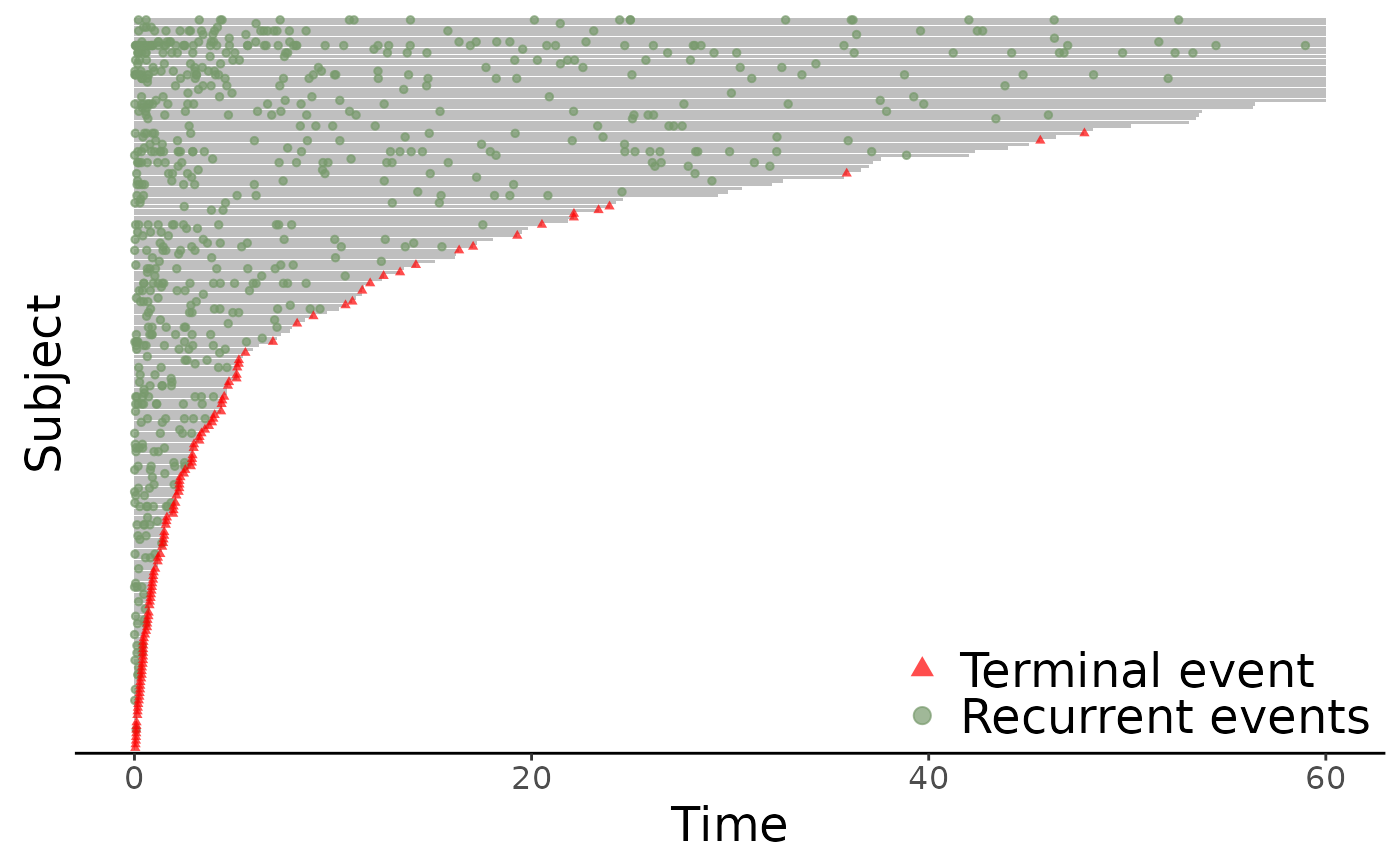plot(reObj, event.result = "decreasing")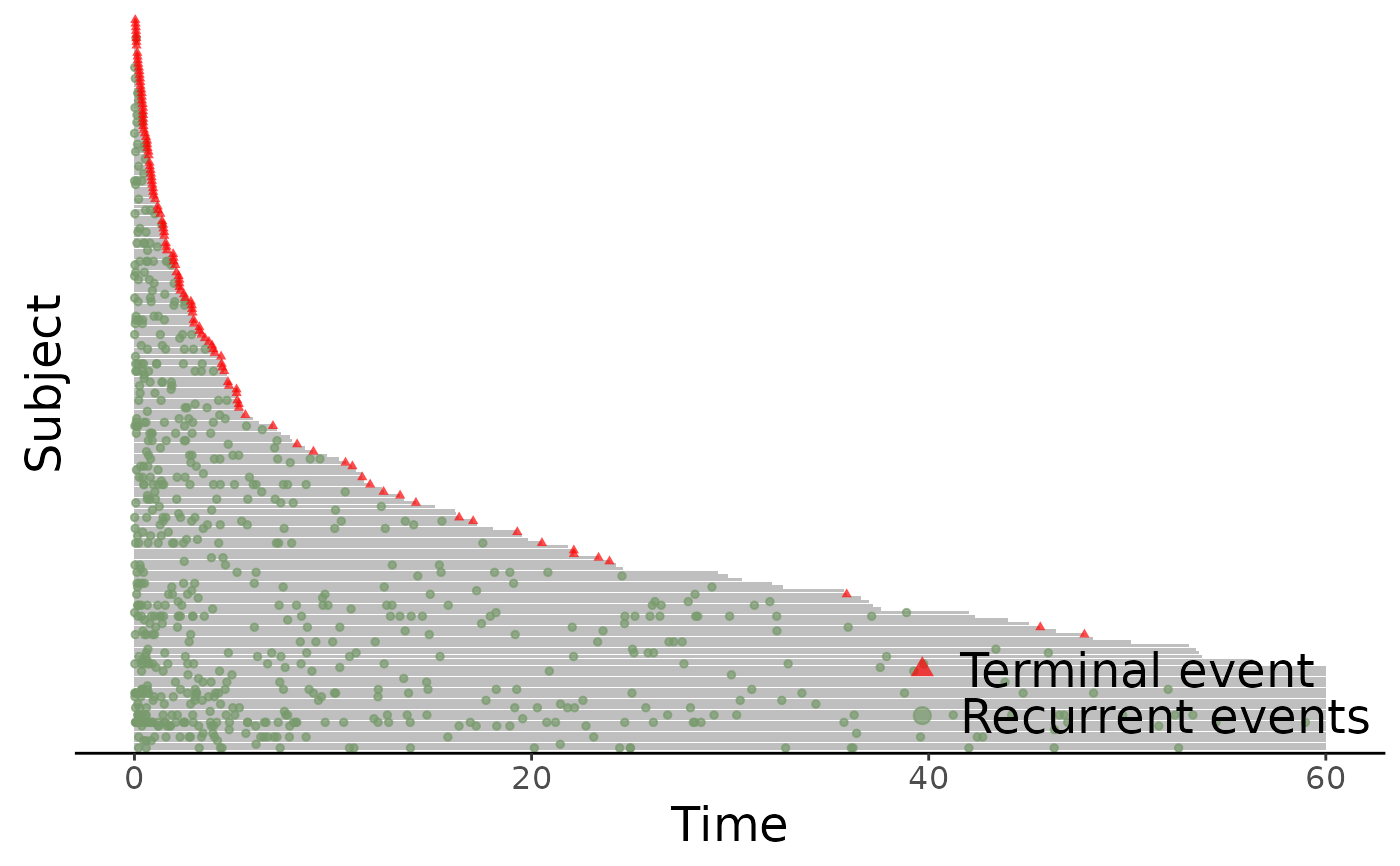## With (hypothetical) multiple event types
simDat$event2 <- with(simDat, ifelse(t.stop > 10 & event > 0, 2, event)) reObj2 <- with(simDat, Recur(t.start %to% t.stop, id, event2, status)) plot(reObj2)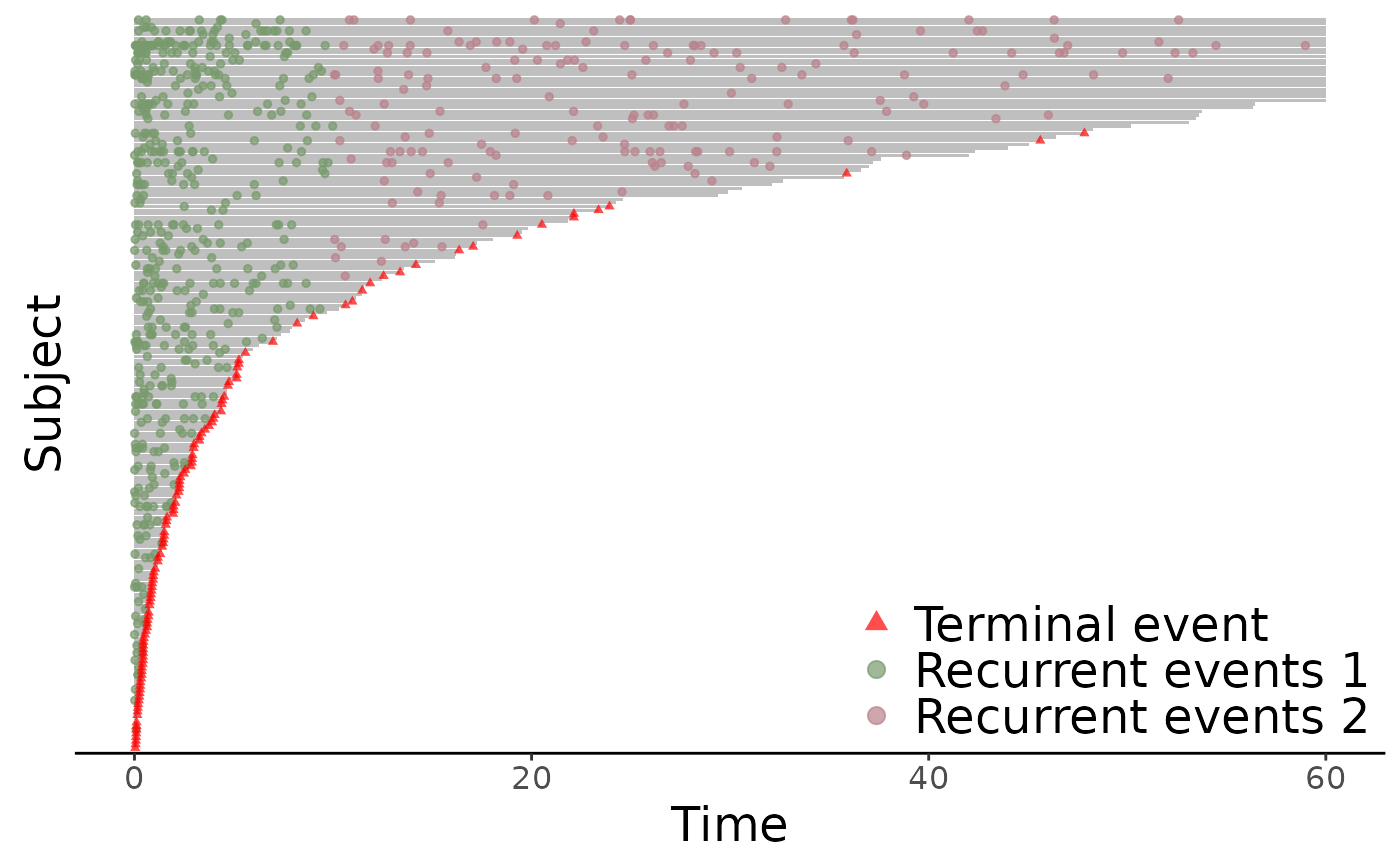## With (hypothetical) calendar times simDat2 <- simDat simDat2$t.start <- as.Date(simDat2$t.start + simDat2$x2 * 5, origin = "20-01-01")
simDat2$t.stop <- as.Date(simDat2$t.stop + simDat2\$x2 * 5, origin = "20-01-01")
reObj3 <- with(simDat2, Recur(t.start %to% t.stop, id, event, status))
plot(reObj3, event.calendarTime = TRUE)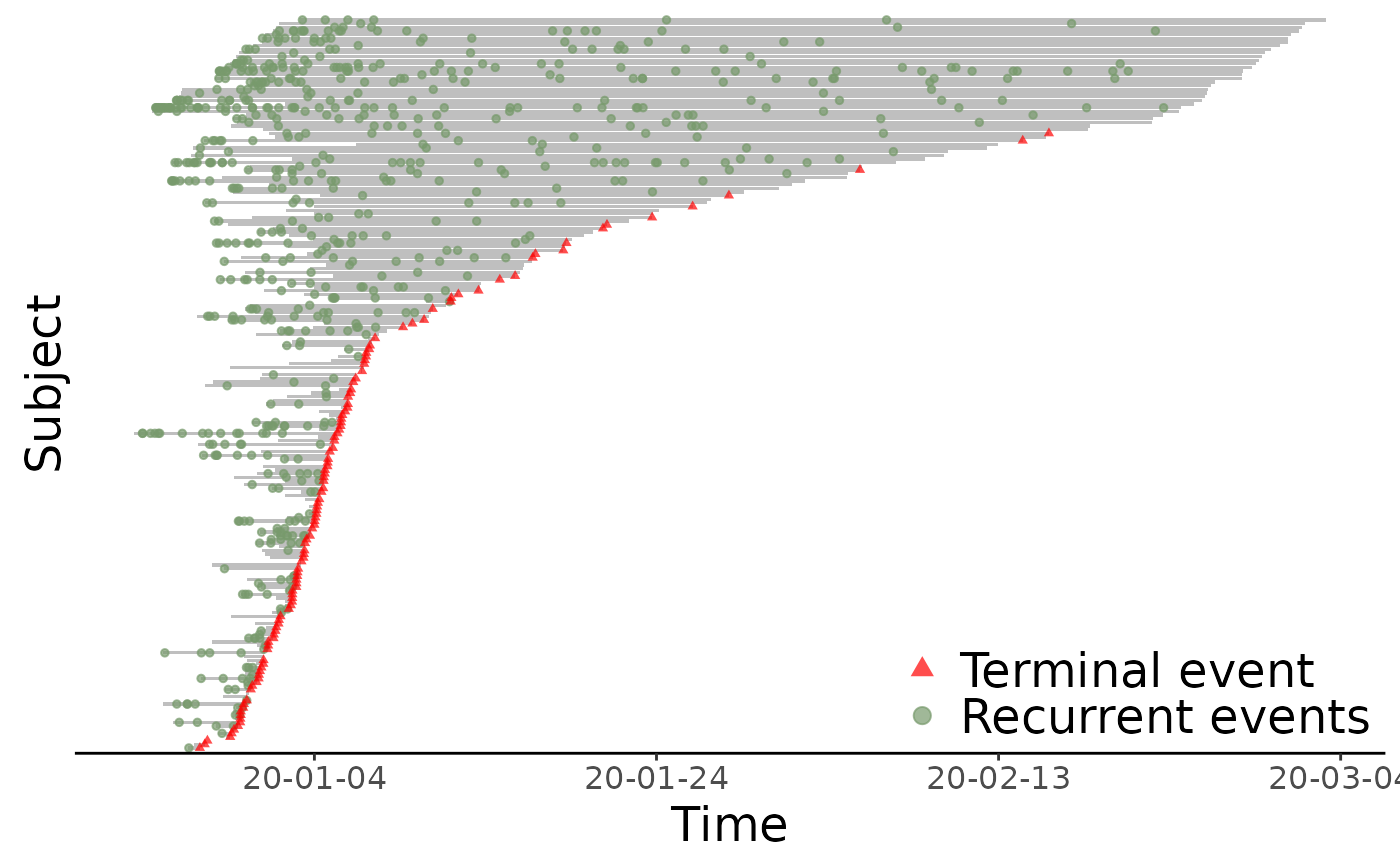## MCF plots
plot(reObj, mcf = TRUE)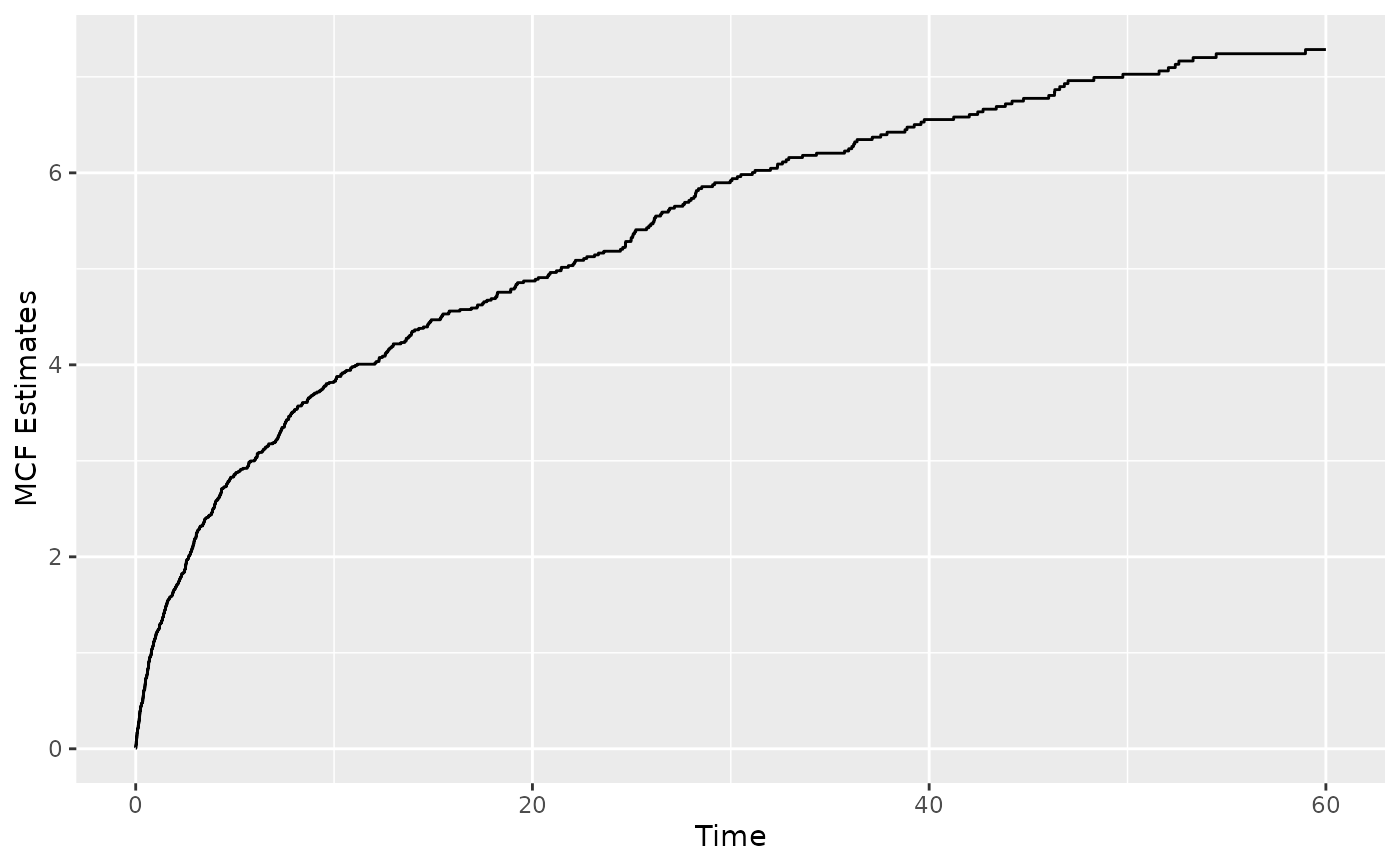plot(reObj, mcf = TRUE, mcf.adjustRiskset = FALSE)